# I have 18 ones, 15 hundreds, 15 tens, and 8 thousands. What number am I?Maxine

# Solution-

1. Start by ones, the value given is 18 of ones. So,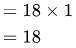1. Proceed with 15 of hundreds. Which will give us,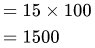1. The next step is 15 of tens. Thus,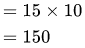1. We have 8 of thousands. Therefore,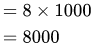The sum of all the answers will give us,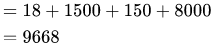Therefore, 18 ones, 15 hundreds, 15 tens, and 8 thousand will give us 9668.

# Similar problems

Question - I have 180 ones, 152 hundreds, 11 tens, and 2 thousands. What number am I?

Solution-

1. Start by ones, the value given is 180 of ones. So,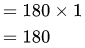1. Proceed with 152 of hundreds. Which will give us,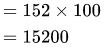1. The next step is 11 of tens. Thus,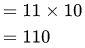1. We have 2 of thousands. Therefore,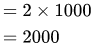The sum of all the answers will give us,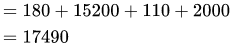Therefore,180 ones, 152 hundreds, 11 tens, and 2 thousand will give us 17490.

Question- I have 11 ones, 12 hundred, 13 tens, and 6 thousand. What number am I?

Solution-

1. Start by ones, the value given is 11 of ones. So,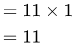1. Proceed with 12 of hundreds. Which will give us,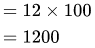1. The next step is 13 of tens. Thus,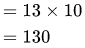1. We have 6 of thousands. Therefore,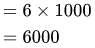The sum of all the answers will give us,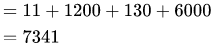Therefore, 11 ones, 12 hundred, 13 tens, and 6 thousand will give us 7341.

### Need homework help now?

tutors online

Related Questions
##### (t) in °C of the pot of soup at time t (in The initial temperature of a pot of soup is 80°C. The temperature minutes) satisfies the differential equation dT di T-20 10 (a) Solve the differential equation, and find an explicit expression for the function (). By separating variables, integrating and manipulating arbitrary constants, . We are also told that so that and so . ...
Notes
Me

OR

Don't have an account?

Join OneClass

Access over 10 million pages of study
documents for 1.3 million courses.

Join to view

OR

By registering, I agree to the Terms and Privacy Policies
Just a few more details

So we can recommend you notes for your school.